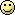View Single Post

Quote:
I am somewhat interested in this simple to build optimized diffuser, but unconvinced. Have you any predictions for other angles of incidence?

EDIT: I just noticed the last pictures, and they do.

But I am still wondering where the optimization is. Is it the frequency domain performance, ease of construction, or something else?
Glad you're interested in the underlying science / math.

I have predictions for 3 angles of incidence (0 degrees, -25 degrees, -45 degrees), as well as a diffuse field (random angles of incidence). More predictions are in the download area.

Details of the optimization system are given in the diffuser design thesis, which you can download here.
• Chapter 3 outlines the design problem.
• Chapter 5 explains the diffusion quality measurement methods.
• Chapter 7 explains the optimization algorithm.

The Design by Optimization System
The design system is basically an efficient trial an error algorithm that uses physical modelling to test the trials, and evolutionary optimization (artificial intelligence based on natural selection) to minimize the error (i.e., converge on a solution).

The process used an integer genetic algorithm to find candidate designs (trial) and evaluated the performance of candidates using a finite difference time domain (FDTD) scattering simulation. The performance of each candidate design was evaluated using a diffusion coefficient based on the "standard error", which is an important quantity used in statistics (see Chapter 5 to see how the diffusion coefficient was calculated). The objective was to minimize the difference between the diffusion coefficient and "ideal diffusion". I.e., minimize the error.

New designs were generated and tested by the system, and using trial and error feedback the system converged to a design that meets the design objectives given below (see Chapter 7 of the thesis for visuals of the convergence process).

Design Objectives
These were the optimization objectives:

The successful diffuser design had to satisfy the following criteria:

1. It must be shallower than existing profiled diffusers, with comparable or better broadband diffusion performance.
2. It should be modular, and designed to disperse sound optimally when modules are arranged an array.
3. It must be economical to manufacture.
4. The geometry must not pose obvious hazards to human safety.
5. The design method should utilize high quality scattering predictions, but it should not require a custom boundary element implementation (Finite difference time domain was used instead).
6. Optimization must produce a suitable design within a reasonable solution time.
7. The design should be suited for application on the back wall of a small-to-medium sized recording studio control room, where the purpose is to disperse first reflections from a hypothetical sound wave arriving at zero degrees incidence.
8. The design method should also be applicable to solving a larger problem: optimizing a surface for uniform scattering of an incident wave from any direction.

The designs you see were optimized for an incident sound wave at zero degrees, but they've also been tested at other incident angles. They performed quite well at all angles tested.

Optimization Frequencies
A Quote from section 7.2 of my thesis, "The Lean Optimization of Acoustic Diffusers":

"Optimization was performed across a range of frequencies that roughly span the 10dB-bandwidth of the excitation pulse. This will be called the diffusion band. 12 discrete frequencies were chosen for optimization, distributed quasi-randomly in the diffusion band. The response spectrum was characterized by a 4096 point FFT (fast fourier transform), and the optimization frequencies were the FFT bins centered on {102, 250, 449, 574, 700, 824, 949, 1102, 1250, 1450, 1700 and 1950 Hz}. This sparse set of FFT bins was chosen in an effort to clearly define the optimization objective, aid in visualizing progress and speed up convergence.

When the goal is to optimize for twelve specific frequencies (rather than all frequencies), the disparity between good solutions and average solutions is higher and results can be compared more meaningfully. Moreover, Cox found that optimization at seven frequencies  was sufficient to achieve good dispersion over the entire bandwidth."

To emphasize the importance of the lower-mid frequencies, the range 400-1250 Hz received the highest density of optimization frequencies. Another option is to optimize across all FFT bins in the diffusion band, assigning relative weights to each bin when solving Eq. (5.2).

Does this answer your questions? I realize I just unleashed a bunch of technical jargon so please let me know if anything is not clear!